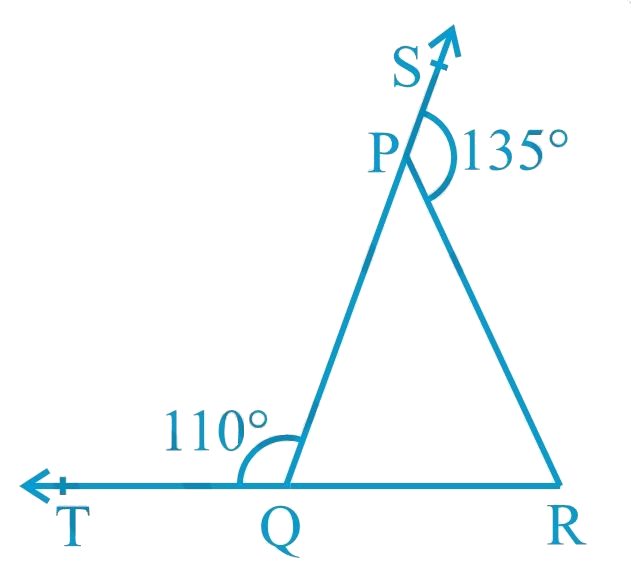# Ex.6.3 Q1 Lines and Angles Solution - NCERT Maths Class 9

Go back to  'Ex.6.3'

## Question

In Fig. below, sides $$QP$$ and $$RQ$$ of $$∆ PQR$$ are produced to points $$S$$ and $$T$$ respectively. If $$\angle SPR = 135^\circ$$ and $$\angle PQT = 110^\circ$$ , find $$\angle PRQ$$.## Text Solution

What is known?

$$\angle SPR = 135^\circ$$ and $$\angle PQT = 110^\circ$$

What is unknown?

$$\angle PRQ$$

Reasoning:

As we know the linear pair axioms:

If Non-common arms of two adjacent angles form a line, then these angles are called linear pair of angles and the sum of the linear pair is $$180^\circ$$.

If the sum of two adjacent angles is $$180^\circ$$ then the two non-common arms of the angles form a line.

Angle sum property of a triangle:

Sum of the interior angles of a triangle is $$360^\circ$$.

Steps:

Given,

$$\angle SPR = 135^\circ$$ and $$\angle PQT = 110^\circ$$

\begin{align}\angle SPR + \angle QPR &= 180^\circ \\ {\text{By linear pair axiom}} \\\\135^\circ + \angle QPR &= 180^\circ \\\angle QPR &=180^\circ\! -135^\circ \\\angle QPR &= 45^\circ.... {\rm{ }}\left( {\rm{i}} \right)\end{align}

\begin{align}\angle PQT + \angle PQR &= 180^\circ \\\begin{array}{I}(\text{By linear pair axiom}) \end{array}\\\\110^\circ + \angle PQR &= 180^\circ \\\angle PQR &= 180^\circ - 110^\circ \\\angle PQR &= 70^\circ..\left( {{\rm{ii}}} \right)\end{align}

Now,

\begin{align}\angle PQR + \angle QPR + \angle PRQ &= 180^\circ\\ (\text{Angle sum } \text{property of a}&\text{ triangle}) \\\\70^\circ + {\rm{ }}45^\circ + \angle PRQ &= 180^\circ\\{\rm{}}\left[ {{\rm{from }}\left( {\rm{i}} \right){\rm{ and }}\left( {{\rm{ii}}} \right)} \right]\\\\\angle PRQ &\!=180^\circ\!\! -\!115^\circ \\\angle PRQ& = 65^\circ \end{align}

Learn from the best math teachers and top your exams

• Live one on one classroom and doubt clearing
• Practice worksheets in and after class for conceptual clarity
• Personalized curriculum to keep up with school